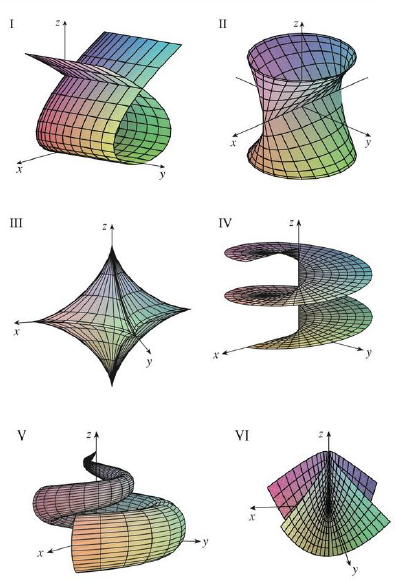Chapter 16.6, Problem 14E

Chapter
Section
Textbook Problem

Match the equations with the graphs labeled I–VI and give reasons for your answers. Determine which families of grid curves have u constant and which have v constant.14. r(u, v) = uv2 i + u2v j + (u2 − v2) kTo determine

To match: The equation r(u,v)=uv2i+u2vj+(u2v2)k with the graphs labeled I-VI in the textbook and to explain the reason for the combination.

Explanation

Given data:

The given vector equation as follows.

r(u,v)=uv2i+u2vj+(u2v2)k

The graphs are labeled in the textbook from I to VI.

Write the vector equation as follows.

r(u,v)=uv2i+u2vj+(u2v2)k

Write the parametric equations from the vector equation as follows.

x=uv2,y=u2v,z=u2v2 (1)

Determination of grid curves when u is constant:

Consider the parameter u as constant u0 .

Substitute u0 for u in equation (1),

x=u0v2,y=u02v,z=u02v2

From the expression y=u02v find the parameter v as follows.

v=(1u02)y

Substitute (1u02)y for v in the expression x=u0v2 ,

x=u0[(1u02)y]2=(1u03)y2

As u0 is considered as constant, consider (1u03) as a constant k .

Therefore, the expression x=(1u03)y2 is written as follows.

x=ky2 (2)

The expression represents the equation of parabola with the axis as x-axis.

Find the v2 from the expression x=u0v2 .

v2=(1u0)x

Substitute (1u0)x for v2 in the expression z=u02v2 ,

z=u02(1u0)x (3)

From equation (2) and (3), each grid curve corresponds to u=u0 (constant), that lies in the plane z=u02(1u0)x and the projection of each grid curve onto the xy-plane is a parabola with the axis as x-axis

Still sussing out bartleby?

Check out a sample textbook solution.

See a sample solution

The Solution to Your Study Problems

Bartleby provides explanations to thousands of textbook problems written by our experts, many with advanced degrees!

Get Started

Find more solutions based on key concepts